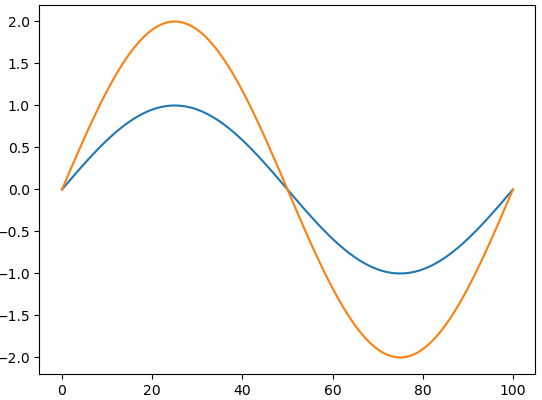# JuliaPro采用PyPlot画图问题

using PyPlot
x = Array(0:100)
y = 2sin.(2pi*x/100)
plot(x,y)
gcf()https://www.codenong.com/52175184/

ENV[“PYTHON”] =“c:\full\path\to\anaconda\bin\python”
using Pkg
Pkg.build(“PyCall”)
using PyPlot